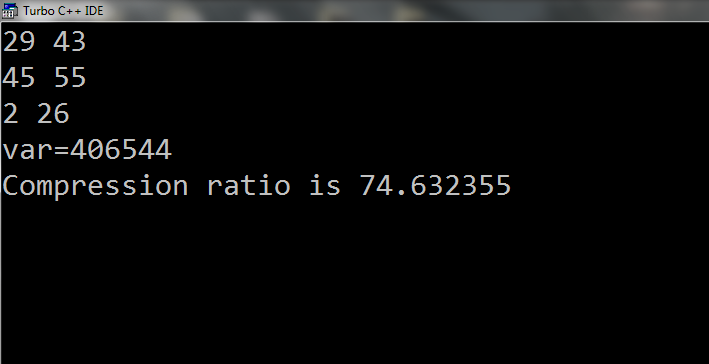# C code to calculate Compression Ratio

New network program in C to calculate Compression Ratio. Compile and Run on Turbo C software. Code is given below:

#include<stdio.h>
#include<conio.h>
void main()
{
char *str="the jamia millia islamia and the jamia milliya islamia are different";
int count=0,t,temp=0,i,var=0,var1,start,end,j,k,l,m=0,n;
float comp=0.0;
clrscr();
i=0;
l=strlen(str);
while(str[i]!='\0')
{
j=i+1;
while(str[j]!='\0')
{
temp=0;
if(str[i]==str[j])
{
start=j;
count=0;
while(str[i]==str[j])
{
count++;
i++;
j++;
temp=1;
}
end=j-1;
if(count>=3)
{
m++;
printf("%d %d\n",start,end);
var=var+end-start+1;
count=0;
getch();
}
}
else
{
if(temp!=1)
j++;
}
}
if(temp!=1)
i++;
}
printf("%d %d",m,var);
var1=((l-var)*9)+(m*14);
printf("\nvar=%d",var1);
t=l*8;
n=var1;
comp=(float)n*100/(float)t;
printf("%d",t);
printf("\nCompression ratio is %f",comp);
getch();
}

//Output Of the above program:-Result of compression ratio

For more c programs related to Network, See the Network label. Share and comment to improve this blog.

Related Programs:-

1.2.